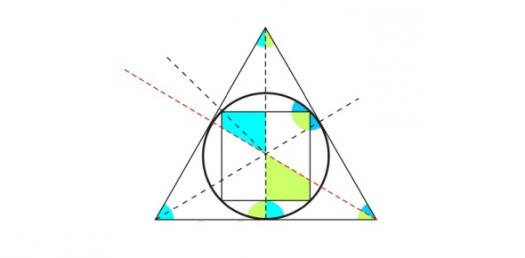# Figurat Dhe Trupat Gjeometrike

Approved & Edited by ProProfs Editorial Team
At ProProfs Quizzes, our dedicated in-house team of experts takes pride in their work. With a sharp eye for detail, they meticulously review each quiz. This ensures that every quiz, taken by over 100 million users, meets our standards of accuracy, clarity, and engagement.
| Written by Valbona
V
Valbona
Community Contributor
Quizzes Created: 1 | Total Attempts: 263
Questions: 10 | Attempts: 263Settings• 1.

### Shuma e këndeve të trekëndëshit është

• A.

90

• B.

360

• C.

180

C. 180
Explanation
The sum of the angles in a triangle is always 180 degrees. Therefore, the correct answer is 180.

Rate this question:

• 2.

### Koni është

• A.

Shumëfaqesh

• B.

Trup në rrotullim

• C.

Figurë gjeometrike

B. Trup në rrotullim
Explanation
The given answer "Trup në rrotullim" is correct because the phrase "Koni është" translates to "Cone is" in English. A cone is a three-dimensional geometric shape that has a circular base and tapers to a point called the apex. It can be visualized as a solid object with a curved surface that rotates around an axis, forming a shape similar to an ice cream cone. Therefore, "Trup në rrotullim" accurately describes the geometric shape of a cone.

Rate this question:

• 3.

### Topi ka formën e :

• A.

Rrethit

• B.

Cilindrit

• C.

Sferes

C. Sferes
Explanation
The correct answer is "Sferes" because the question is asking for the form of a "topi ka," which translates to "shape of a hat" in English. Out of the given options, a hat is most commonly associated with the shape of a sphere.

Rate this question:

• 4.

### Trekëndëshat klasifikohen :

• A.

Sipas llojit te kendeve

• B.

Sipas gjatesise se brinjeve

• C.

Te dyja se bashku

C. Te dyja se bashku
Explanation
The correct answer is "Te dyja se bashku" which means "Both together" in English. This suggests that the classification of treks can be based on both the type of terrain and the length of the trails. This means that when categorizing treks, both the type of path and the distance covered are taken into consideration.

Rate this question:

• 5.

• A.

Po

• B.

Jo

A. Po
• 6.

### Drejtkëndeshi është figurë gjeometrike

• A.

E vërtetë

• B.

E gabuar

A. E vërtetë
Explanation
The given statement "Drejtkëndeshi është figurë gjeometrike" is true. A drejtkëndesh (rectangle) is a geometric figure with four sides and four right angles. It is a quadrilateral with opposite sides that are equal in length and parallel to each other. Therefore, the correct answer is "E vërtetë" (True).

Rate this question:

• 7.

### Katrori është :

• A.

Trup gjeometrik

• B.

Figure gjeometrike

• C.

Ekuacion

B. Figure gjeometrike
Explanation
The correct answer is "Figure gjeometrike." This answer is correct because "katrori" translates to "square" in English, which is a type of geometric figure. Therefore, "figure gjeometrike" is the most appropriate option to describe a square.

Rate this question:

• 8.

### Paralelogrami ka :

• A.

Dy cifte brinjësh paralele

• B.

Këndet e barabarta

• C.

Ķatër brinjë të barabarta

A. Dy cifte brinjësh paralele
Explanation
The given answer "Dy cifte brinjësh paralele" means "Two pairs of parallel sides" in Albanian. This explanation suggests that a parallelogram has two pairs of sides that are parallel to each other. It does not mention anything about equal angles or equal sides, which are not necessarily true for all parallelograms.

Rate this question:

• 9.

### Cili katërkëndësh ka katër brinjë me gjatësi të barabartë dhe asnjë kënd të drejtë?

• A.

Katrori

• B.

Drejtkendeshi

• C.

Rombi

• D.

Trapezi

C. Rombi
Explanation
A square is a type of quadrilateral with four equal sides and four right angles. In the given question, it is mentioned that the quadrilateral has four equal sides and no right angles. A square cannot have no right angles, so it cannot be the correct answer. A rectangle is a type of quadrilateral with four right angles, but it is not mentioned in the question that the angles are right angles. Therefore, a rectangle cannot be the correct answer. A rhombus is a type of quadrilateral with four equal sides, but it does not necessarily have right angles. Since the question does not mention anything about the angles, a rhombus can be the correct answer. A trapezoid is a quadrilateral with at least one pair of parallel sides, which is not mentioned in the question. Therefore, a trapezoid cannot be the correct answer.

Rate this question:

• 10.

### Cili është ndryshimi mes një prizmi dhe një piramide?

• A.

Nuk ka ndryshim: varet nga forma e bazës.

• B.

Piramida ka vetëm një kulm ndërsa prizmi ka të njëjtën prerje me planin gjatë gjithë gjatësisë së tij.

• C.

Piramidat janë trekëndore, kurse prizmat janë katrorë

• D.

Një prizëm është rrethor.Back to top